# Everything Looks The Same

## Katie Steckles

The symbol for equality, =, has been in use for hundreds of years. Its invention is often credited to Welsh physician and mathematician Robert Recorde, who used the notation as early as 1557 – although Italian manuscripts from half a century earlier have also been found to use a similar symbol. Regardless of its origins, it is clear that equality as a concept is important to mathematicians. The unambiguous equating of two things to say they are the same as each other is fundamental to many mathematical ideas and methods.

You might be used to encountering equality in simple numerical and algebraic equations – in specifying a value, like x = 4, or in setting up a relationship between variables, like x = y + 7 – or even just in writing out the steps in a calculation, like 2 + 3 = 5. But there’s another kind of equality, which mathematicians consider at a deeper fundamental level. It’s not just numbers and variables that can be equal to each other: any kind of mathematical structure or object can possess isomorphism.

## Isomorphin’ Time

The notion of isomorphism crops up all over mathematics. If two objects are isomorphic, they can be seen to have the same underlying structure, or be able to map onto each other completely. It doesn’t mean the two objects are exactly the same – they may differ wildly in their nature or complexity – but an isomorphism means we can find a structure within each shape which looks identical. Various symbols are used to denote isomorphism, including sometimes the classic = sign, as well as a version of it with a tilde above, ​`≅`, often used in formal notation.

Way back in 2019, I wrote about the 15 Game – a game of picking numbers between 1 and 9 to try to obtain a total of 15 using exactly three numbers. This showed an interesting correspondence between two seemingly unrelated games – if you write the numbers 1-9 in a magic square, in which each row, column and diagonal contains three numbers that sum to 15, the challenge of finding a winning set while preventing your opponent from finding one is exactly the challenge of winning a game of noughts and crosses. The isomorphism between these two games is defined by the magic square.

We can also consider isomorphisms between mathematical structures, rather than two games. Consider the set of symmetries of an equilateral triangle. This includes all the ways to rotate and reflect the triangle which will give back the same shape – rotation by 60, 120, and 360 degrees, and reflection in three different axes which pass through the centre of the triangle. There are six different symmetries (including rotation by 360 degrees, which can be considered to be trivial as it doesn’t change anything and might as well be a rotation by 0 degrees).

This set of symmetries forms a mathematical group, which means we can combine them to produce other symmetries – a reflection combined with a rotation gives the same effect as reflection in one of the other axes. As a group, this has a structure isomorphic to another familiar mathematical idea: the set of ways to shuffle a stack of three cards.

Consider three distinct cards, and the number of ways to shuffle them. We can imagine choosing an ordering, and for the bottom card on the stack we have three options; then once we’ve chosen a card, there are two options for the middle card, and one remaining card which must go on top. This means there are 3 × 2 = 6 ways to order the cards – and it turns out these correspond exactly to our six orientations of the triangle. The diagram below shows how some of these symmetries can be matched with card orderings.

## A Hanoi-ing Coincidence

Another nice example of a correspondence I’ve found is related to the Towers of Hanoi puzzle. The puzzle involves a set of different-sized round flat stones, each with a hole in the centre, which are placed on a stick in height order.

The challenge of the puzzle is to move the tower of stones from one stick to another; there’s a third stick which you are allowed to use as a temporary holding position, but you’re never allowed to place a larger stone on top of a smaller one. There’s a playable version here at MathIsFun (If you have never seen this puzzle, or would like a chance to re-familiarise yourself with it, I suggest you do so now as I will be giving away some aspects of the solution below).

While for a given number of stones there are many ways to solve this puzzle, there’s an optimal number of moves which can serve as a goal for maximum efficiency, and be considered the best solution. For three stones, it can be achieved in 7 moves, which are as follows:

This is the minimal set of moves to get three stones across to the other end; if we number the three stones 1, 2 and 3 (with 1 being the smallest and 3 being the largest), we can encode this pattern by listing the stone that gets moved each time (and in almost all cases, there’s only one option for where to move it to; in the rare cases there is a choice, it turns out to be equivalent. In this run, the only decision is where to put the smallest stone at the start, and choosing differently would just result in the final stack being on the middle peg).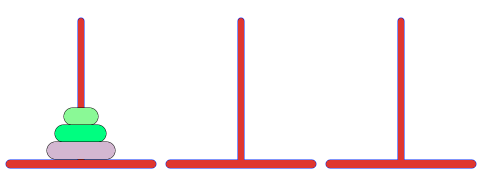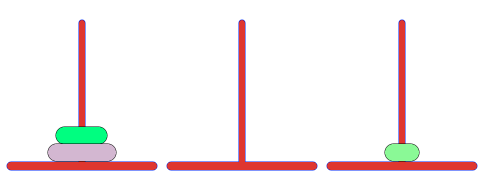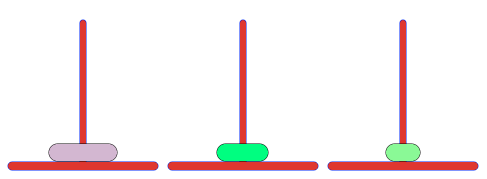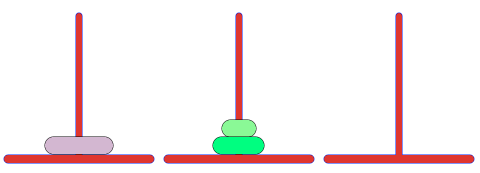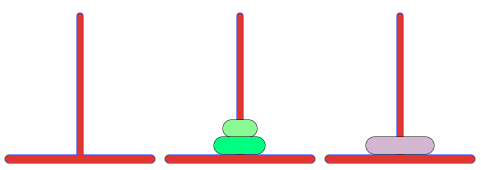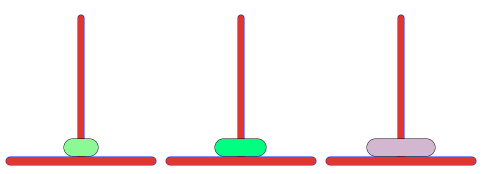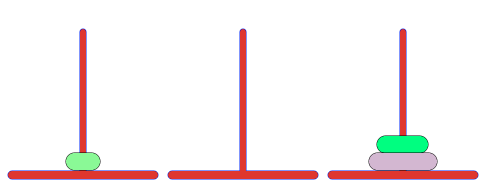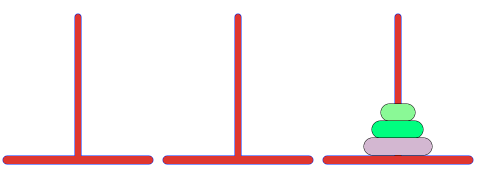For three stones, the pattern of stone moves is: 1 2 1 3 1 2 1. This can be extended to give a solution for four stones – by completing this pattern once, to move the first three stones off the largest one, then moving the largest into its final position, then moving the three back onto it using this sequence again. This gives: 1 2 1 3 1 2 1 4 1 2 1 3 1 2 1. If you look carefully, you can see this is exactly what we did at the lower level, to move the 1 and 2 off the three using ‘1 2 1’, then move the 3, then put them back on again.

In fact, optimal solutions for any number of stones can be derived by repeating the chunk of moves corresponding to the solution for n-1 stones on either side of a single move of the nth stone. The smallest stone (1) moves every other turn, bouncing around while the others move into place, and the largest stone waits its turn and makes one big move to its final destination. A satisfying pattern! Surely unique to this particular problem?

Well, no. This pattern crops up in many other places, including the path to visit all the corners of an n-dimensional cube (where the numbers correspond to which of the n dimensions you move in), and my personal favourite: the least significant digit of binary numbers. If you write numbers in binary and mark the position of the 1 that’s furthest to the right in each number, this will follow the same pattern.

## I Can Hasse

One final isomorphism I find deeply enjoyable comes from the notion of partial orderings. There are some objects in mathematics which can be totally ordered – for example, numbers have a well-defined ordering, and given any two distinct numbers I can tell you which is less than or greater than the other. Given a collection of sets, this becomes more difficult.

For example, the collection of all possible sets containing elements x, y, and z contains eight sets: the empty set, with none of them in and denoted ; the single-element sets {x}, {y} and {z}; the two-element sets {x,y}, {y,z} and {x,z}, and the set containing all three: {x,y,z}. This construction is called the power set of the set {x,y,z}, and in general the power set of a finite set will always contain 2k sets, where k is the number of elements in your original set.

If we wanted to put an ordering on this collection of sets, it is sometimes possible – the set {x,y} somehow must be ‘bigger than’ the set {x} since one is contained in the other. But which is bigger out of {x,y} and {x,z}? And is {x} bigger than {y}? They’re not equal, since they contain different elements, but is an ordering possible?

The notion of partial ordering means we can define an ordering which gives a rule for some pairs of elements but not others. A Hasse diagram, named after mathematician Helmut Hasse, encodes this information graphically, with an arrow pointing from any set to one which contains it: